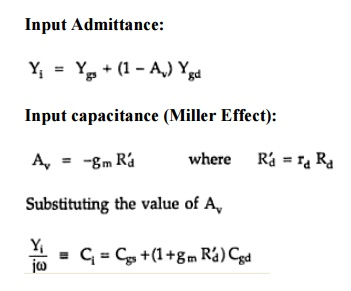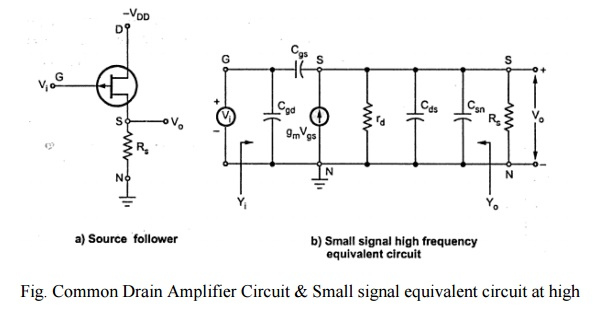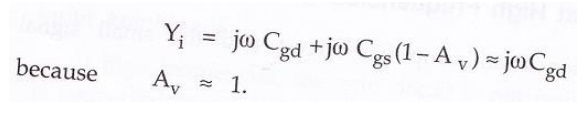Home | | Electronic Circuits II | High frequency analysis of MOSFET

# High frequency analysis of MOSFET

1. Common source amplifier at high frequencies 2. Common Drain Amplifier at High Frequencies

High frequency analysis of MOSFET:

## 1. Common source amplifier at high frequencies:This increase in input capacitance Ci over the capacitance from gate to source is called Miller effect.

This input capacitance affects the gain at high frequencies in the operation of cascaded amplifiers. In cascaded amplifiers, the output from one stage is used as the input to a second amplifier. The input impedance of a second stage acts as a shunt across output of the first stage and Rd is shunted by the capacitance Ci.

From above figure, the output impedance is obtained by looking into the drain with the input voltage set equal to zero. If Vi = 0 in figure, rd , Cds and Cgd in parallel. Hence the output admittance with RL considered external to the amplifier is given by,## 2. Common Drain Amplifier at High Frequencies:Fig. Common Drain Amplifier Circuit & Small signal equivalent circuit at high frequencies.

### Voltage gain:

The output voltage Vo can be found from the product of the short circuit and the impedance between terminals S and N. Voltage gain is given by,Input Admittance Yi  can be obtained by applying Miller‘s theorem to Cgs. It is given by,